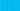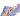PricingResources

# What is the double declining balance (DDB) depreciation method?

Written by

Businesses have multiple methods at their disposal to account for depreciation. One option is the double declining balance depreciation method. Here’s a closer look at how this method is calculated and when it should be used.

## What is the double declining depreciation method?

Sometimes called the reducing balance method, the double declining depreciation method offers a way to account for an asset’s reduction in value over time. While methods like straight-line depreciation use a consistent rate of depreciation for each year of an asset’s lifespan, accelerated depreciation methods like DBB show a steep drop in the first years of the asset’s life. After this, there will be increasingly smaller depreciation expenses recorded over the later years of the lifespan.

## When to use the double declining depreciation method

Business expenses must be recorded in the same accounting period as revenue earned due to the expenses. This is a fundamental tenet of the generally accepted accounting principles (GAAP) for any public company. So, if a company purchases an asset that it plans to use over several years, it wouldn’t deduct the entire cost as a business expense up front. This is because it intends to earn revenue as a result of that asset over a longer timeframe. The price must be deducted over the asset’s lifespan – but what happens when the asset depreciates rapidly in value?

As the name suggests, the double declining depreciation calculation depreciates an asset’s value twice as rapidly as the declining balance method. It’s most commonly used for assets that plummet in value early on, particularly high-tech equipment that will quickly become obsolete.

## Double declining depreciation formula

There are a couple of different ways to approach the double declining depreciation formula:

Or

## How to calculate double declining depreciation

As you can see from the formulas above, you’ll need to know a few key numbers to calculate double declining depreciation. This includes the asset’s beginning cost or book value, its useful life, and its ultimate salvage value. Here are a few steps you can use in this double declining depreciation calculation:

1. Calculate the asset’s beginning book value. What did you pay for it?

2. Calculate the asset’s useful life. How long do you plan to use it?

3. Estimate the asset’s salvage value. What will it be worth at the end of its lifespan?

4. Subtract salvage value from book value to determine the asset’s total depreciable amount.

5. Calculate the annual depreciation rate.

6. Multiple the beginning book value by twice the annual depreciation rate.

7. Subtract the annual depreciation expense from the book value to calculate the final value.

8. Repeat the process until the salvage value is reached.

## Double declining depreciation example

What does this calculation look like in practice? Imagine the following double declining depreciation example:

Business A purchases a delivery vehicle for \$20,000. It’s expected to last for 10 years. At the end of this 10-year period, the vehicle will only be worth \$2,000 which is its salvage value. \$20,000 minus \$2,000 equals \$18,000, which would be divided by 10 for a loss of \$1,800 each year using the straight-line depreciation method.

However, under the double declining balance method, the 10% is doubled so that the vehicle loses 20% of its value each year.

• In the first year, it would lose 20% of the book rate, \$20,000, or \$4,000.

• In the second year, it would lose 20% of \$16,000, or \$3,200.

• In the third year, it would lose 20% of \$12,800, or \$2,560.

• In the fourth year, it would lose 20% of \$10,240, or \$2,048.

• In the fifth year, it would lose 20% of \$8,192, or \$1,638.40.

This would go on until it reaches its final salvage value. As you can see, after only five years, or half of its lifespan, the vehicle would have plummeted in value from \$20,000 to \$6,553.60 for expense reporting purposes.

## Tax advantages of using double declining depreciation

There are some advantages to choosing double declining depreciation. By choosing to accelerate depreciation and take on a higher expense in the earlier years of your asset’s lifespan, net income is transferred to later years of its use. Taxes are pushed back accordingly.

While the total expense remains the same over the life of the asset, the expenses are timed differently depending on the depreciation method you choose. Because depreciation costs are tax-deductible, you would pay lower income tax in the early years of asset use and higher taxes later on.

## We can help

GoCardless helps you automate payment collection, cutting down on the amount of admin your team needs to deal with when chasing invoices. Find out how GoCardless can help you with ad hoc payments or recurring payments.## Interested in automating the way you get paid? GoCardless can help

Contact sales

Sales

Contact Sales

+1(415) 523-2279

Support

help@gocardless.com

+1 (628) 241-0044